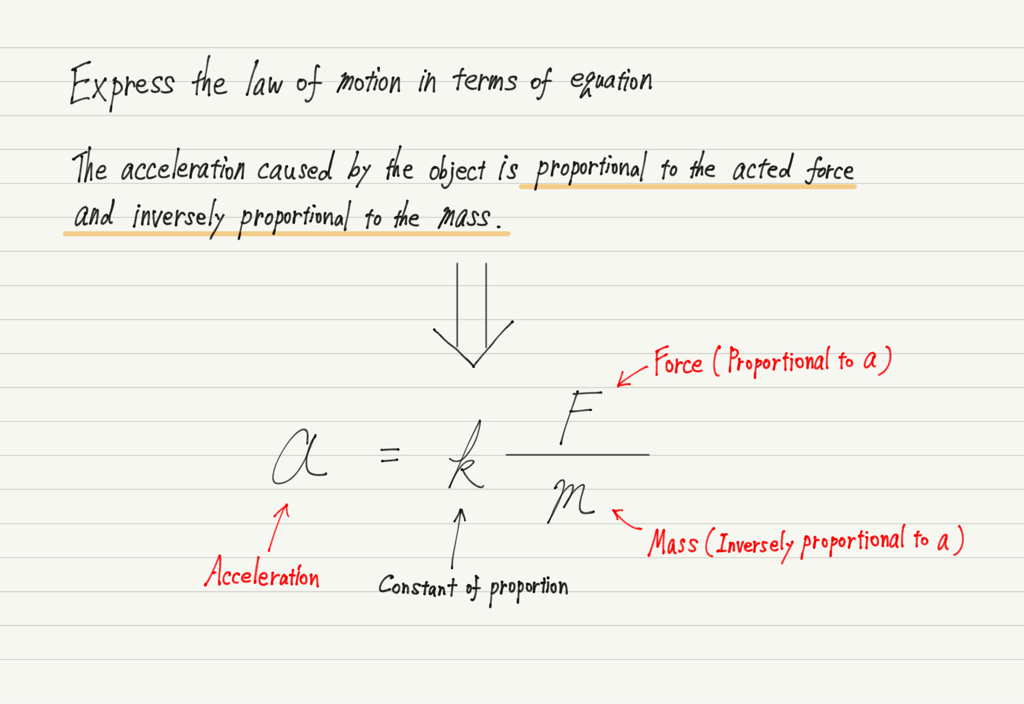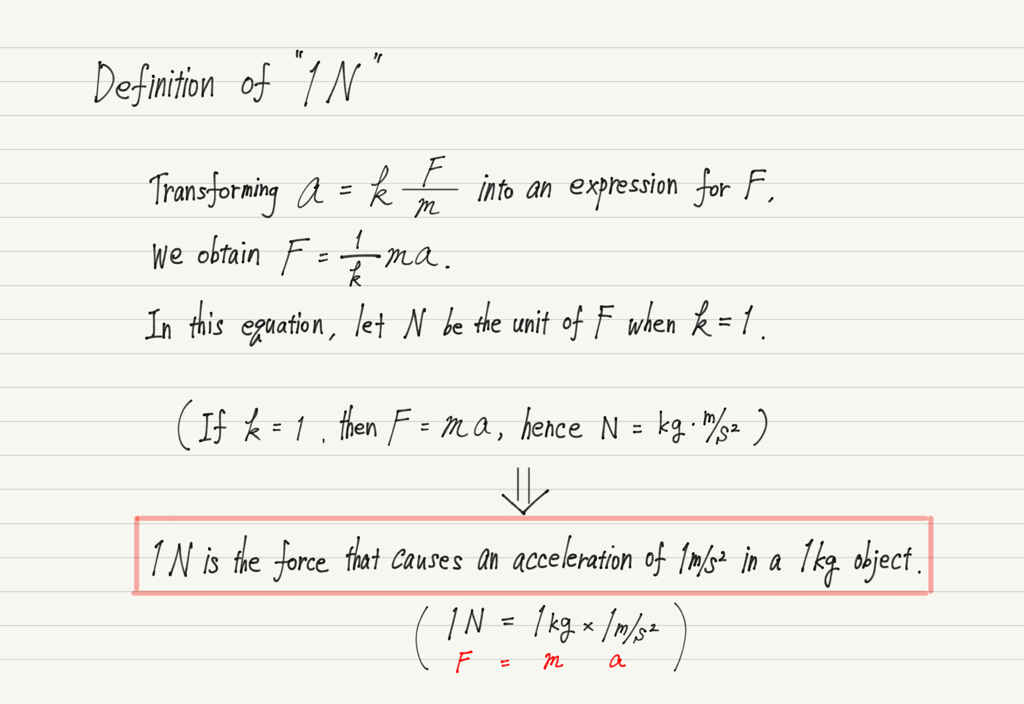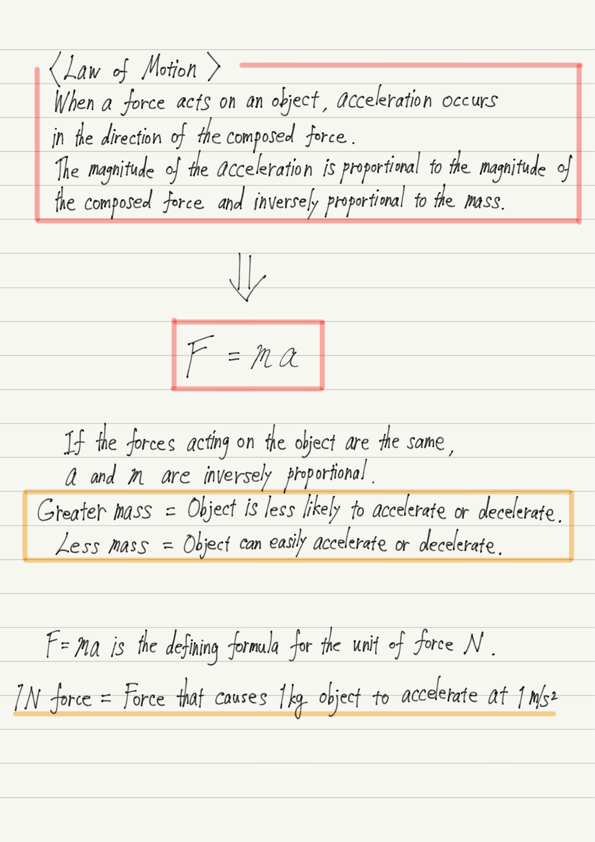# Law of Motion

This time we will study the second law of motion.
The second law of motion, also known as the “law of motion,” is often referred to as the “second law” as it is.

First, a review. The first law of motion (the law of inertia) is true when the forces acting on an object are equilibrated.
However, the forces are not always equilibrated.

What happens to an object when the forces are not equilibrated? The second law of motion shows us this.

## Deciphering the law of motion

Let us introduce the content of the law of motion.

“When a force is acted an object, the object experiences an acceleration in the direction of the force.
The magnitude of the acceleration is proportional to the magnitude of the force and inversely proportional to the mass of the object.”

The “force” in the first half represents the composed force. For example, if an object is being pulled by 5 N to the right and 2 N to the left, the composed force will be 3 N to the right, which means that the object will accelerate to the right.

There are several important aspects of this law, the most important being “acceleration”.

We said that the second law of motion shows what happens to an object when the forces are not equilibrated, and if the forces are not equilibrated, the object will naturally start moving.
Anyone knows this even if he or she has not studied physics.

However, although it is correct up to the point where “a stationary object starts to move when a force is acted”, many people misunderstand the rest of the story!

“The greater the force acted, the faster the object moves, so speed is proportional to force.”

This is a huge mistake!

## Force and Speed are not proportional

Let us consider why this is wrong.

If the magnitude of the force is proportional to the speed, then doubling the force should double the speed, tripling the force should triple the speed, and so on.
In the same way, if the force is set to zero, the speed is also zero.

Now, think of a bicycle. At first glance, the proportional relationship seems to be correct, since the harder you pedal, the faster the bicycle goes.

But when you stop pedaling (i.e., the force acted is zero), does your speed become zero?

It won’t happen!

There is no such thing as a bicycle that stops the moment you stop pedaling!
If you stop rowing, you should still be going at the same speed you were just before you stopped.

Now, according to the law of inertia, “when no force is acted, a moving object is in uniform linear motion.
If we assume that the force is proportional to the speed, it contradicts the law of inertia.

So how should we think about it? Actually, the answer lies hidden in the law of inertia!

According to the law of inertia, if a force is not acted, the state of motion will not change.
In other words, “the state of motion changes when a force is acted.
A change in state of motion means that a stationary object starts to move, and a moving object speeds up or slows down.

This is exactly what acceleration is all about!

Newton discovered that force is not related to velocity, but to acceleration

## Objects with large mass are hard to stop

Using the law of motion, correctly describe the motion of a bicycle.

Pedaling → Positive acceleration is generated → Bicycle goes faster.

Stop pedaling → No acceleration because no force is exerted → Bicycle moves in a uniform linear motion.

Braking → Negative acceleration → Bicycle decelerates.

The latter part, “acceleration is inversely proportional to mass,” is also important.
This means that the greater the mass, the smaller the acceleration produced by a force of the same magnitude.

In other words, a large mass makes it difficult to accelerate and difficult to decelerate.
When the mass is small, it is easy to accelerate and easy to decelerate.

Applying this to daily life, for example, when driving a bicycle or a car, the more luggage or passengers you have, the harder it is to slow down (i.e., the longer it takes to stop), so it is good to keep this in mind for safe driving.

## Express the law of motion in equations

Since the law of motion is law that state the proportional relationship between acceleration and force and the inverse proportional relationship between acceleration and mass, they can be expressed as equations.This formula is not used as is, so there is no need to memorize it.
Just make sure that you can write it as an equation because it is proportional and inversely proportional.

## Definition of 1 N

Finally, the unit of force “N (newton)” can be defined here.

You may have heard that the magnitude of gravity acting on a 100 g object is approximately 1 N, but that is not accurate.
In fact, the unit 1 N is defined using the law of motion.Now that we have determined 1 N, let’s continue to treat the proportionality constant k of the law of motion as 1!

This lecture has been a long one, so I will end this session with a summary of the content of the lecture up to this point.

## Summary of this lecture## Next Time

The laws of motion are still deep, so we’ll continue this next time!

https://www.yukimura-physics.com/entry/dyn-f22

error: Content is protected !!